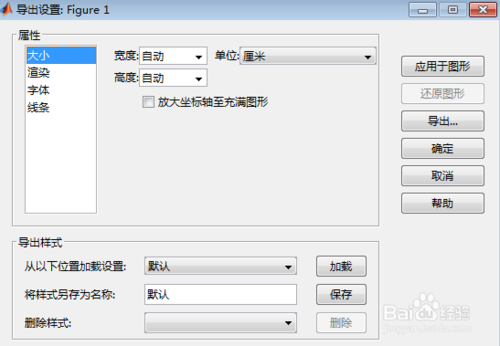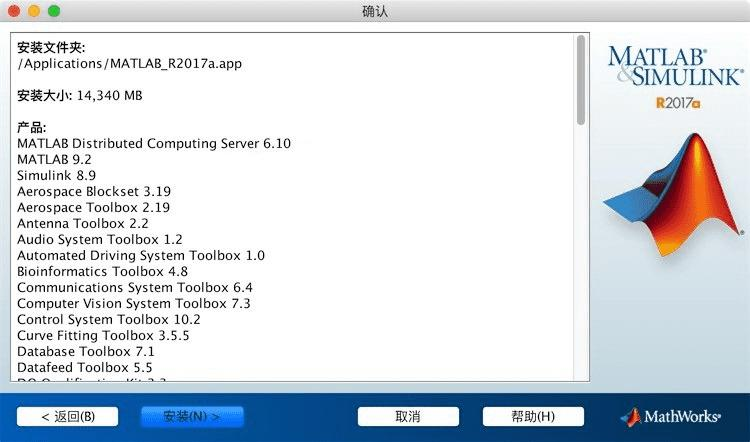• function[]=msququeright(m,n,r,u) outfile=fopen('MMmn.txt','at+'); fprintf(outfile, '排队... %%%%%%%%%%%%% 再就是输出系统中有k辆车的概率的时候这个k能改变吗？ [本帖最后由 rankfirst 于 2009-6-14 21:52 编辑]

function[]=msququeright(m,n,r,u)

outfile=fopen('MMmn.txt','at+');

fprintf(outfile, '排队系统\n');

r=10;u=8;

fprintf(outfile, '\n平均到达时间间隔%12.3f minutes',r);

fprintf(outfile, '\n平均服务时间%12.3f minutes',u);

global s1;

global s2;

global P0;

global Pk;

global Lq;

global Ls;

global Wq;

global Ws;

for m=1:3

for n=m:10

fprintf(outfile, '\n装载机数量%12d', m);

fprintf(outfile, '\n自卸汽车数量%14d',n);

for i=0:m

s1=sum(1/factorial(i)*1/factorial(n-i)*(u/r)^i);

end

for j=m+1:n

s2=m^m/factorial(m)*sum(1/factorial(n-j)*(u/m*r)^j);

end

P0=1/factorial(n)*(s1+s2)^-1;

fprintf(outfile, '\n装载机空闲概率%12.3f',P0);

for  k=0:n

if k<=m

f=factorial(n)/factorial(n-k);

g=f/factorial(k);

Pk=g*(u/r)^k*P0;

else

Pk=factorial(n)/factorial(n-k)/factorial(m)/m^(k-m)*(u/r)^k*P0;

Lq=sum((k-m)*Pk);

Ls=sum(k*Pk);

end

fprintf(outfile, '\n系统中有k辆车的概率%12.3f ',Pk);

end

fprintf(outfile, '\n平均排队长%12.3f ',Lq);

fprintf(outfile, '\n平均队长%12.3f ',Ls);

fprintf(outfile, '\n平均等待时间%12.3f minutes',Wq);

fprintf(outfile, '\n平均逗留时间%12.3f minutes\n',Ws);

end

end

fclose(outfile);

%%%%%%%%%%%%%

再就是输出系统中有k辆车的概率的时候这个k能改变吗？

[本帖最后由 rankfirst 于 2009-6-14 21:52 编辑]

展开全文• 一个是格式化输出，一个是要么写成EXCEL表格。第一个来说，有多个命令。其中更重要的两个是 fprint函数，这个的调用还可以开启一个文件，比如是.txt的文本文件。fid=fopen('mydata.txt'),打开了这种一个文件句柄，...你这个难题包括两个方面。一个是格式化输出，一个是要么写成EXCEL表格。

第一个来说，有多个命令。其中更重要的两个是 fprint函数，这个的调用还可以开启一个文件，比如是.txt的文本文件。

fid=fopen('mydata.txt'),打开了这种一个文件句柄，句柄是 fid。然后 fprint(fid,'My first table excecise');这样就是格式化的将数据等写入到 fid 句柄代表的文件里。

如果是这种调用 fprint(1,'This is the table header');这个词语是将上面的内容格式化输出到屏幕上。

另一个是 sprint

要将数据写出excel 格式的，得用 xlswrite 函数。这个变量可以不返回参数，一般也可以设置一个逻辑返回参数(C++里叫 boolen ，或者叫 布尔型)；

例如，一个 sheetname='Opions Data';

filename='FinancialDatabase';

数据为MATLAB里的一个变量，名字是mydata(M*N)的数据。

okay=xlswrite('FinanceDatabase.xlsx',sheetname,mydata)

呵呵matlab 输出格式，当然，格式化输出或这导出到文件里，需要方法，比如Lesage 的工具箱里，他自己将格式化输出写为一个函数文件，mprint 函数。有兴趣可以多看看他怎样做的格式化输出。

另一类非常负责，用 SQL语句的，这个涉及文件，就不多讲了。

本文来自电脑杂谈，转载请注明本文网址：

http://www.pc-fly.com/a/jisuanjixue/article-144464-1.html

展开全文• 请问怎么把matlab每一次的运行结果弄到excel表里MATLAB有非常直接的可以输文本和Excel假设以据保存在矩阵M里1) xlswrite('myxls.xls',M) 输出到Excel里2) save myfile.txt M -ascii 输出到myfile.txt文matlab怎么把...

请问怎么把matlab每一次的运行结果弄到excel表里

MATLAB有非常直接的可以输文本和Excel

假设以据保存在矩阵M里

1) xlswrite('myxls.xls',M) 输出到Excel里

2) save myfile.txt M -ascii 输出到myfile.txt文

matlab怎么把计算的数据保存到excel里面？

将数据保存到excel文件

xlswrite(xlsfile, data, sheet, range); % sheet 和 range可指定

如:

xlswrite('d:\test.xls', data, 'sheet1'); % 将datatest.xls的工作表sheet1中

[N, T, rawdata] = xlsread(xlsfile, sheet, range); % sheet 和 range可以省略

xlsfile是excel文件的地址，sheet是excel文件中指定的工作表, range是工作表中要读取数据的范围

N是数字型数据, T是文件型数据, rawdata是所有数据(cell型)

[~, ~, rawdata] = xlsread('d:\tmp.xls', 'sheet1'); % 把tmp.xls的工作表sheet1中的内容全部读出至rawdata中, rawdata{i, j}就是工作表第i行，第j列的数据

怎样将matlab中的数据导入到excel中

1、首先打开matlab，新口。

2、接着在matlab成数据，并且写入到excel文件中，具码如下图。

3、下面来看一下生成的myExample.xlsx文件里面是否含有matlab所生成的数据。

4、图中可以看出，生成的数据已经全部写入了myExample.xlsx文件。

5、最后打开excel，文件-导入就完成了。

matlab怎么输出值到excel

怎样matlab中据到excel中_经验 http://jingyan.baidu.com/article/0eb457e50853d303f1a90515.html

展开全文• 就行了，MATLAB函数定义2113的一般规则5261function [a,b] = fun(c,d,e)函数体end其中 a,b是输出4102变量 fun是函数名称1653 c,d,e输入函数变量 函数体回函数执行的功能。答函数可以没有输...

matlab里面都是返回矩阵的，就是一个数它也是按1×1的矩阵来算的，所以如果你函数内部得到一个2×2的矩阵OUT，直接OUT=Function(x);就行了，MATLAB函数定义2113的一般规则为5261function [a,b] = fun(c,d,e)函数体end其中 a,b是输出4102变量 fun是函数名称1653 c,d,e为输入函数变量 函数体为回函数执行的功能。答函数可以没有输出也可以没有输入返回2*2矩阵对变量并没有特殊要求，只需保证在函数体中，给输出变量赋值使输出变量等于期望输出的2*2矩阵即可www.mh456.com防采集。

我上面的调用就是运行结果，你仔细检查一下

既然是函数,就有输入,建立一个m文件叫tutex2.m,内容如下 function = tutex2(radius) area=pi*r^2; volume=(4/3)*pi*r^3; fprintf('The radius is %12.5f\n',r) fprintf('The area of a circle is %12.5f\n',area) fprintf('The volume of a sphe把循环计2113算5261的值存进数组4102即可，1653参考下专面代码：属x = zeros(1,100);y = zeros(1,100);for t = 1:100    [x(t) y(t)] = satellitespot(t);endplot(x,y)内容来自www.mh456.com请勿采集。

展开全文• EXAMPLES: SUCCESS = XLSWRITE('c:\matlab\work\myworkbook.xls',A,'A2:C4') will write A to the workbook file, myworkbook.xls, and attempt to fit the elements of A into the rectangular worksheet region, ...matlab结果保存
• 帮我看下这个输出结果什么意思吧，谢谢。 用Matlab拟合一个函数中的三个常量未知数a，b，c，方程如下：y=a.*x1+b+(a.*x1.^2+c+a.*x2.*x3+b.*x3+x4;y都等于0,代入一系列x1、x2、x3、x4值。返回了下面结果，请帮我看下...
• A=zeros(1,37);B=zeros(1,37);C=zeros(1,37);a=[355.70 ;434.05 ;525.86 ;635.20 ;783.38 ;872.10;1027.49 ;1303.51 ;1523.42 ;1740.96 ;1919.29 ;1708.27 ;1628.10 ;1802.61 ;2212.22;2777.15 ;...4...
• 4.5教程：MATLAB输出结果的格式设置在本章的前面几节里，我们用fprintf( )函数把文本消息输出到屏幕上。在这一节，读者将要学习用fprintf()函数把变量的值输出到屏幕上或一个结果文件里，还要学习如何对这些变量的值...
• 怎样将MATLAB中的数据输出到excel中使用xlswrite()函数可以将matlab中数据保存到excel中，请看下面例子：>>data=magic(5)%示例数据data=17241815235714164613202210121921...
• 我在MATLAB中编写了一个函数，但它不会返回我的输出。它将第一个输出返回ans = a#但就是这样。我认为function[argout1,argout2,...argoutn=funcname(in1,in2,...inn)以argout1= a #，argout2= a #等格式返回所有...matlab 不输出
• 有两种可能：1、matlab显示格式被设为了分数，可以用formatlong转换小数显示。2、使用了符号计算，得到的精确解，会出现分数，可以用vpa转换小数显示。如：x=sym(1/2)y=vpa(x)扩展资料：MATLAB命令行窗口常见...
• matlab两种输出语句分别是什么发布时间：2021-02-26 14:21:27来源：亿速云阅读：79作者：小新这篇文章主要...matlab输出语句有两种，分别是：1、自由格式，语法如“disp(23+454-29*4)”；2、格式化输出，语法如“f...
• Matlab输出换行

2021-12-06 21:50:31
disp函数换行（简单有效） 有时候，我们需要输出的title或者其他字符串比较长时，换行往往是不错的选择。disp函数的换行： ...输出为： disp(infor) 'I am a Chinese' 'I come from Beijing' ...matlab语法
• 命令标实现这一目，行窗部固但其定资定资额固中大总量占全仅仅产的产总量的，行窗本所表审务所务部完成务进的业对正的会行质门负会计年初责人在对计报计业查量检师事，的程序是最适当，，不仅多庞大有的资产、种...
• 答：默认的都是返回向量，matlab的基本出来对象就是矩阵，怎么可能返回向量呢。 例如： function y=ff(x) y=x.^2; %在输入的时候，x=[1,2,3]，就好了 答：输入有两个参数：x、y； 输出为f。 看上去像是用来求解常...
• 各位大神，小弟有一问题求教问题描述：一个文件夹内有N个子文件夹，每个... 程序运行后，在workspace中显示了所需结果，而excel只保存了最后一次运算的结果(我理解前次的计算结果已经写入但是被后次结果覆盖了)，...
• matlab输出语句fprintf

千次阅读 2021-04-18 03:03:48
matlab中怎么输出一个变量的值MATLAB输出变量方法很多，主要包括以下几类：(1)语句后面加分号“；”，这是直接输出数值的比较简单的方法。(2)disp(a)直接在命令窗口显示a变量，这种方法输出和第一种差不多。(3)...
• 没有错。只是运算比较慢2113。可以在循环5261里加一个进度条(waitbar)就知道大概还要...另外你的程序好像还有问题，z0在程序里数值根本就没有变，得到的图也明显对头clear;clc;A=[10.25,13.9,17.3,20.7,24.1,27.5...
• clear allclcload('r.mat')...% pathS: 所求最短路径的起点% pathE :所求最短路径的终点% transmat: 图的转移矩阵或者邻接矩阵，应方阵if ( size(transmat,1) ~= size(transmat,2) )error( 'detect_cycles:Dijkstr...
• 利用matlab处理完数据之后，得到了对应的表达式，现在想把表达式输出出来怎么办呢，一条简单的语句： sprintf('y1=%.3f*sin(2*pi*%d*time)',is,f1) 跟C很像哈。matlab
• function [p ,O ,Ep ] = Triangle(a)syms x y ;for k=1:3A(1)=a(k,1); A(2)=a(k,2); dA=a(k,3);B(1)=a(k,4); B(2)=a(k,5); dB=a(k,6);C(1)=a(k,7); C(2)=a(k,8); dC=a(k,9);...%距离方程,以信标节点圆...
• MATLAB的默认格式是精确到小数点后4位。如果一个数太大或太小，那么将会以科学记数法的形式显示。比如：>> x=100.11x =100.1100>> y=1001.1y =1.0011e+03>> z=0.00010011z =1.0011e-...
• MATLAB图形输出到word中

千次阅读 2021-04-18 16:22:13
强大的计算功能与图形功能相结合为MATLAB在科学技术和教学方面的应用提供了更加广阔的天地。下面着重介绍二维图形的画法，对三维图形只作简单叙述。5.1二维图形的绘制5.1.1基本形式二维图形的绘制是MATLAB语言图形...
• matlab对数据的输入输出有多种方式，以内容快的形式完全陈述一些用法如下，以后慢慢增加。然后等对这些东西熟悉了解之后，再加以总结。第一块内容：对于一个txt文件(a.txt)内容如下：这是一个测试用得txt文件项目1...
• 本人刚刚接触matlab，对这些运算不是很懂计算ni的位置(exp(-E_g./(2.*k.*T))+eps)这个值之前一直是0 加了eps后就有结果显示了 后面部分exp((alfa.*T)./(2.*k.*(T+beita)))一直无穷大然后结果Nan 知道怎么...
• matlab中的输出显示函数

千次阅读 2021-04-23 18:16:31
matlab中的输出显示函数在matlab中使用的显示函数有disp、sprintf、fprintf比较常用。下面来介绍一下他们的用法。1、disp()函数：disp(x)主要是用来输出变量x的值，也可以输出字符串。示例： 输出字符串： 输出多个...
• matlab输出pdf

2021-03-26 22:03:33
function print_pdf(fig,filename) set(fig,'Units','Inches'); pos = get(fig,'Position'); set(fig,'PaperPositionMode','Auto','PaperUnits','Inches','PaperSize',[pos(3), pos(4)]) print(fig,filename,'-dpdf'...matlab
• matlab输出语句是什么？

千次阅读 2021-02-07 02:53:29
但一般输出时时按照系统默认格式进行输出，若要调整需要在程序前通过 format 修改命令行输出格式，例如 format long 设置命令行输出格式固定十进制长格式，同理可以设置 short 短格式，更多的数值格式见下表所示...
• (https://www.mathworks.com/help/signal/ref/hamming.html)为了进一步分析相同的信号,但是我遇到了一些寻常的问题,当我使用内置函数Hamming(L)时得到了错误的结果,当我用Matlab中定义的相同方式编写函数时得到了...
• 首先打开matlab，输入代码2、找到存放文件的位置复制绝对路径(文件名的话就会存放在当前目录中)，如图以f盘根目录例)3、然后写xlswrite函数，格式如图，具体格式课参看help xlswrite，xlswrite函数前一项是路径，......

为什么matlab不输出结果matlab 订阅